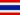# 4.1 Panorámica de programas de hoja de cálculo

• Apuntes
• Revisión por temaWAI P. 0 0 Lesson 4 - Introduction to Spreadsheet Programs 4.1 - Overview of Spreadsheet Programs Overview of Spreadsheet Programs Using a spreadsheet program, such as Excel 2007, you can store and modify data. You can also perform various mathematical calculations, such as addition, subtraction, and multiplication. The interface of Excel 2007 includes the following parts: Workbook and worksheets. A workbook is a file created in Excel 2007. A workbook can contain one or more worksheets and related items. An Excel 2007 workbook contains three worksheets. A worksheet is a single spreadsheet in a workbook. You can add or delete worksheets in a workbook as required. Rows and columns. The horizontal divisions in a worksheet are called rows. Each row is identified by a number. For example, the first row in a worksheet is 1. Columns are the vertical divisions in a worksheet. Each column is identified by a letter. For example, the first column in a worksheet is A. Cell. A cell is formed by the intersection of a row and a column. The highlighted rectangular border formed around an active cell is called a cell pointer. An active cell is the cell in which you are working currently. There can be only one active cell at a time. You can use a cell to store and display different types of data such as text, numbers, or formulas. Each cell in a worksheet is identified by a cell address. A cell address is made of the column letter and row number of the cell. For example, the cell formed by the first column and first row has the cell address A1. The cell address indicates the exact location of a cell in a worksheet. Status bar. This bar provides information about the present status of work in the worksheet. For example, if you are not working on the worksheet, the status bar displays the message "Ready". When you click in a blank cell to enter data, the status bar displays the message "EnterWAI P. 0 0 Explain the Enter key?Janeya Z. 0 0 interesting.